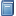Buchempfehlung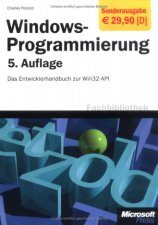Windows-Programmierung. Das Entwicklerhandbuch zur WIN32-API
"Der" Petzold, das über 1000 Seiten starke Standardwerk zum Win32-API - besonders nützlich u. a. bei der GUI-Programmierung in FreeBASIC! [Mehr Infos...]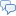FreeBASIC-Chat
Es sind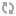Benutzer im FreeBASIC-Chat online.
(Stand:  )

# fb:porticula NoPaste

## GSM_ACM.bi

 Uploader: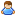ThePuppetMaster Datum/Zeit: 19.08.2009 08:39:52
Hinweis: Dieser Quelltext ist Bestandteil des Projekts GSM_ACM, zu dem es auf FreeBASIC-Portal.de eine Projektseite gibt.

```'#################################################################################################################################### '#################################################################################################################################### ' GSM_ACM.bi --- GSM Command Modul '#################################################################################################################################### '#################################################################################################################################### ' 2009 By.: /_\ DeltaLab's Germany - Experimental Computing ' Autor: Martin Wiemann ' Version: 1.00.0 - 14.08.2009 - 12:17:35 '#################################################################################################################################### ' Licence: "Do What The Fuck U Want" '#################################################################################################################################### '#################################################################################################################################### '#################################################################################################################################### #Include once "vbcompat.bi" '#################################################################################################################################### Enum GSMACM_GuruCode     GSMACM_NoError                      = 1     GSMACM_Unknown                      = 0     GSMACM_CantOpenDevice               = -1     GSMACM_ComIDInUse                   = -2     GSMACM_UnknownDeviceID              = -3     GSMACM_CommandError                 = -4     GSMACM_CommandTimeout               = -5     GSMACM_CantReadReturnData           = -6     GSMACM_SMSIDNotFound                = -7     GSMACM_InvalidPIN                   = -8 End Enum '------------------------------------------------------------------------------------------------------------------------------------ Enum GSMACM_NetworkState_Enum     GSMACM_NS_NoLogin_NoNetsearch       = 0     GSMACM_NS_Login_Homenet             = 1     GSMACM_NS_NoLogin_Netsearch         = 2     GSMACM_NS_NoLogin_LoginDenied       = 3     GSMACM_NS_Unknown                   = 4     GSMACM_NS_Login_AnotherNet          = 5 End Enum '------------------------------------------------------------------------------------------------------------------------------------ Enum GSMACM_SMSState_Enum     GSMACM_SMSS_Unknown                 = 0     GSMACM_SMSS_RecUnread               = 1     GSMACM_SMSS_RecRead                 = 2     GSMACM_SMSS_StoUnsent               = 3     GSMACM_SMSS_StoSent                 = 4     GSMACM_SMSS_All                     = 5 End Enum '#################################################################################################################################### Type GSMACM_INT_Type     V_Next                              as GSMACM_INT_Type Ptr     V_Prev                              as GSMACM_INT_Type Ptr     V_ComID                             as UByte     V_DeviceID                          as Integer     V_ThreadID                          as Any Ptr     V_ThreadExit                        as UByte     V_Event_SMS                         as Sub (V_DeviceID as Integer, V_SMSID as UInteger, V_CallerID as String, V_ReceiveTime as Double, V_Message as String)     V_Event_Call                        as Sub (V_DeviceID as Integer, V_CallerID as String)     V_Event_NetState                    as Sub (V_DeviceID as Integer, V_NewNetworkState as GSMACM_NetworkState_Enum)     V_Event_BatteryState                as Sub (V_DeviceID as Integer, V_IsLoading as UByte, V_LoadlevelPercent as UByte)     V_DelSMSonEvent                     as UByte End Type Dim Shared GSMACM_INT_F                 as GSMACM_INT_Type Ptr Dim Shared GSMACM_INT_L                 as GSMACM_INT_Type Ptr Dim Shared GSMACM_INT_Mutex             as Any Ptr '#################################################################################################################################### Function GSMACM_INT_GetPtr(V_DeviceID as Integer) as GSMACM_INT_Type Ptr Dim TPtr as GSMACM_INT_Type Ptr = GSMACM_INT_F Do Until TPtr = 0     If TPtr->V_DeviceID = V_DeviceID Then Return TPtr     TPtr = TPtr->V_Next Loop Return 0 End Function '------------------------------------------------------------------------------------------------------------------------------------ Function GSMACM_INT_CheckComIDFree(V_ComID as UByte) as UByte Dim TPtr as GSMACM_INT_Type Ptr = GSMACM_INT_F Do Until TPtr = 0     If TPtr->V_ComID = V_ComID Then Return 0     TPtr = TPtr->V_Next Loop Return 1 End Function '#################################################################################################################################### Function GSMACM_GetGURUCode(V_GuruCode as GSMACM_GuruCode) as String Select Case V_GuruCode     Case GSMACM_NoError:                Return "No error!"     Case GSMACM_Unknown:                Return "Unknown error!"     Case GSMACM_CantOpenDevice:         Return "Can't open device!"     Case GSMACM_CommandError:           Return "Command error! Maybe the device doesn't support this command or a parameter error!"     Case GSMACM_CommandTimeout:         Return "Timeout while sending command and wait for results!"     Case GSMACM_CantReadReturnData:     Return "Can't read or can't understand the result data from the device!"     Case Else:                          Return "Unknown GURU-Code!" End Select End Function '#################################################################################################################################### Function GSMACM_INT_7Bit_Decode(V_Text as String) as String Dim T as String Dim XL as UInteger = Len(V_Text) For X as UInteger = 1 to XL     Select Case V_Text[X - 1]         Case &H00:  T += Chr(&H40)         Case &H01:  T += Chr(&HA3)         Case &H02:  T += Chr(&H24)         Case &H03:  T += Chr(&HA5)         Case &H04:  T += Chr(&HE8)         Case &H05:  T += Chr(&HE9)         Case &H06:  T += Chr(&HF9)         Case &H07:  T += Chr(&HEC)         Case &H08:  T += Chr(&HF2)         Case &H09:  T += Chr(&HC7)         Case &H0B:  T += Chr(&HD8)         Case &H0C:  T += Chr(&HF8)         Case &H0E:  T += Chr(&HC5)         Case &H0F:  T += Chr(&HE5)         Case &H10:  T += Chr(&HCE, &H94)         Case &H11:  T += Chr(&H5F)         Case &H12:  T += Chr(&HCE, &HA6)         Case &H13:  T += Chr(&HCE, &H93)         Case &H14:  T += Chr(&HCE, &H9B)         Case &H15:  T += Chr(&HCE, &HA9)         Case &H16:  T += Chr(&HCE, &HA0)         Case &H17:  T += Chr(&HCE, &HA8)         Case &H18:  T += Chr(&HCE, &HA3)         Case &H19:  T += Chr(&HCE, &H98)         Case &H1A:  T += Chr(&HCE, &H9E)         Case &H1B             If X < XL Then                 Select Case V_Text[X]                     Case &H0A:  T += Chr(&H0C)                     Case &H14:  T += Chr(&H5E)                     Case &H28:  T += Chr(&H7B)                     Case &H29:  T += Chr(&H7D)                     Case &H2F:  T += Chr(&H5C)                     Case &H3C:  T += Chr(&H5B)                     Case &H3D:  T += Chr(&H7E)                     Case &H3E:  T += Chr(&H5D)                     Case &H40:  T += Chr(&H7C)                     Case &H65:  T += Chr(&HE2, &H82, &HAC)                 End Select             End If         Case &H1C:  T += Chr(&HC6)         Case &H1D:  T += Chr(&HE6)         Case &H1E:  T += Chr(&HDF)         Case &H1F:  T += Chr(&HC9)         Case &H24:  T += Chr(&HA4)         Case &H40:  T += Chr(&HA1)         Case &H5B:  T += Chr(&HC4)         Case &H5C:  T += Chr(&HD6)         Case &H5D:  T += Chr(&HD1)         Case &H5E:  T += Chr(&HDC)         Case &H5F:  T += Chr(&HA7)         Case &H60:  T += Chr(&HBF)         Case &H7B:  T += Chr(&HE4)         Case &H7C:  T += Chr(&HF6)         Case &H7D:  T += Chr(&HF1)         Case &H7E:  T += Chr(&HFC)         Case &H7F:  T += Chr(&HE0)         Case Else:  T += Chr(V_Text[X - 1])     End Select Next Return T End Function '#################################################################################################################################### Function GSMACM_INT_SendCMDX(V_DeviceID as Integer, V_CMD as String, R_DD() as String, ByRef R_DC as UInteger, V_Blank as UByte = 0) as GSMACM_GuruCode R_DC = 0 If V_DeviceID = 0 Then Return GSMACM_UnknownDeviceID MutexLock(GSMACM_INT_Mutex) If GSMACM_INT_GetPtr(V_DeviceID) = 0 Then MutexUnLock(GSMACM_INT_Mutex): Return GSMACM_UnknownDeviceID MutexUnLock(GSMACM_INT_Mutex) Dim TPtr as GSMACM_INT_Type Ptr Dim XECall as Sub (V_DeviceID as Integer, V_CallerID as String) Dim T as String Dim T1 as String Dim D as String = Chr(0) Dim XPos as UInteger MutexLock(GSMACM_INT_Mutex) Print #V_DeviceID, "AT+" & V_CMD & Chr(13, 10); Dim XTot as Double = Timer + 1 Dim XTOut as Double = Timer + 10 Dim XDD() as String Dim XDC as UInteger Dim TCalID as String Dim XV as GSMACM_GuruCode Do     Get #V_DeviceID, 0, D     If D <> Chr(0) Then T1 += D     Do         XPos = InStr(1, T1, Chr(13, 10))         If XPos = 0 Then Exit Do         T = Left(T1, XPos - 1)         T1 = Mid(T1, XPos + 2)         If T <> "" Then '           Print ">>>"; Left(T, Len(T)); "<<<"             Do                 XPos = 0                 If Right(T, 1) = Chr(13) Then XPos = 1: T = Left(T, Len(T) - 1)                 If Right(T, 1) = Chr(10) Then XPos = 1: T = Left(T, Len(T) - 1)                 If XPos = 0 Then Exit Do             Loop             If Left(T, 3) <> "AT+" Then                 Select Case UCase(T)                     Case "OK":                  MutexUnLock(GSMACM_INT_Mutex): Return GSMACM_NoError                     Case "ERROR":   R_DC = 0:   MutexUnLock(GSMACM_INT_Mutex): Return GSMACM_CommandError                     Case "+CRING: VOICE"                         TCalID = "" '                       XV = GSMACM_INT_SendCMDX(V_DeviceID, "CLCC", XDD(), XDC) '                       If XV = GSMACM_NoError Then '                           If XDC > 0 Then '                               TCalID = XDD(1) '                           End If '                       End If                         MutexLock(GSMACM_INT_Mutex)                         TPtr = GSMACM_INT_GetPtr(V_DeviceID)                         If TPtr <> 0 Then                             XECall = TPtr->V_Event_Call                             MutexUnLock(GSMACM_INT_Mutex)                             If XECall <> 0 Then XECall(V_DeviceID, TCalID)                         Else: MutexUnLock(GSMACM_INT_Mutex)                         End If                     Case Else                         R_DC += 1                         Redim Preserve R_DD(R_DC) as String                         If V_Blank = 0 Then                             XPos = InStr(1, T, ":")                             If XPos > 0 Then                                 R_DD(R_DC) = Trim(Mid(T, XPos + 1))                             Else: R_DD(R_DC) = T                             End If                         Else: R_DD(R_DC) = T                         End If                 End Select             End If             T = ""         End If     Loop     If XTot < Timer Then Sleep 1, 1: XTot = Timer     If XTOut < Timer Then Exit Do Loop MutexUnLock(GSMACM_INT_Mutex) Return GSMACM_CommandTimeout End Function '------------------------------------------------------------------------------------------------------------------------------------ Function GSMACM_INT_GetSingleCMD(V_DeviceID as Integer, V_CMD as String, ByRef R_Data as String = "") as GSMACM_GuruCode R_Data = "" Dim DD() as String Dim DC as UInteger Dim RV as Integer = GSMACM_INT_SendCMDX(V_DeviceID, V_CMD, DD(), DC) If RV <> GSMACM_NoError Then Return RV If DC = 0 Then Return GSMACM_CantReadReturnData For X as UInteger = 1 to DC     If DD(X) <> "" Then R_Data = DD(X): Exit For Next Return GSMACM_NoError End Function '#################################################################################################################################### Function GSMACM_Get_BatteryState(V_DeviceID as Integer, ByRef R_IsLoading as UByte, ByRef R_LoadlevelPercent as UByte) as GSMACM_GuruCode Dim T as String Dim RV as GSMACM_GuruCode = GSMACM_INT_GetSingleCMD(V_DeviceID, "CBC=?", T) Dim XLLM as UInteger Dim XPos as UInteger If RV = GSMACM_NoError Then     XPos = InStr(1, T, "(")     If XPos > 0 Then         T = Mid(T, XPos + 1)         XPos = InStr(1, T, "(")         If XPos > 0 Then             T = Mid(T, XPos + 1)             XPos = InStr(1, T, "-")             If XPos > 0 Then XLLM = ValUInt(Left(T, XPos - 1))         End If     End If End If RV = GSMACM_INT_GetSingleCMD(V_DeviceID, "CBC", T) If RV <> GSMACM_NoError Then Return RV XPos = InStr(1, T, ",") If XPos <= 0 Then Return GSMACM_CantReadReturnData R_IsLoading = CUByte(ValUInt(Trim(Left(T, XPos - 1)))) If XLLM > 0 Then     R_LoadlevelPercent = CUByte(100 / XLLM * ValUInt(Trim(Mid(T, XPos + 1)))) Else: R_LoadlevelPercent = CUByte(ValUInt(Trim(Mid(T, XPos + 1)))) End If Return GSMACM_NoError End Function '------------------------------------------------------------------------------------------------------------------------------------ Function GSMACM_Get_Time(V_DeviceID as Integer, ByRef R_Time as Double) as GSMACM_GuruCode Dim T as String Dim RV as GSMACM_GuruCode = GSMACM_INT_GetSingleCMD(V_DeviceID, "CCLK?", T) If RV <> GSMACM_NoError Then Return RV If Len(T) < 20 Then Return GSMACM_CantReadReturnData R_Time = DateSerial(ValInt("20" & Mid(T, 2, 2)), ValInt(Mid(T, 5, 2)), ValInt(Mid(T, 8, 2))) R_Time += TimeSerial(ValInt(Mid(T, 11, 2)), ValInt(Mid(T, 14, 2)), ValInt(Mid(T, 17, 2))) R_Time = DateAdd("h", ValInt(Mid(T, 29)), R_Time) Return GSMACM_NoError End Function '------------------------------------------------------------------------------------------------------------------------------------ Function GSMACM_Get_Manufacturer(V_DeviceID as Integer, ByRef R_Manufacturer as String) as GSMACM_GuruCode Return GSMACM_INT_GetSingleCMD(V_DeviceID, "CGMI", R_Manufacturer) End Function '------------------------------------------------------------------------------------------------------------------------------------ Function GSMACM_Get_Model(V_DeviceID as Integer, ByRef R_Model as String) as GSMACM_GuruCode Return GSMACM_INT_GetSingleCMD(V_DeviceID, "CGMM", R_Model) End Function '------------------------------------------------------------------------------------------------------------------------------------ Function GSMACM_Get_Revision(V_DeviceID as Integer, ByRef R_Revision as String) as GSMACM_GuruCode Return GSMACM_INT_GetSingleCMD(V_DeviceID, "CGMR", R_Revision) End Function '------------------------------------------------------------------------------------------------------------------------------------ Function GSMACM_Get_IMEI(V_DeviceID as Integer, ByRef R_IMEI as String) as GSMACM_GuruCode Return GSMACM_INT_GetSingleCMD(V_DeviceID, "CGSN", R_IMEI) End Function '------------------------------------------------------------------------------------------------------------------------------------ Function GSMACM_Get_IMSI(V_DeviceID as Integer, ByRef R_IMSI as String) as GSMACM_GuruCode Return GSMACM_INT_GetSingleCMD(V_DeviceID, "CIMI", R_IMSI) End Function '------------------------------------------------------------------------------------------------------------------------------------ Function GSMACM_Get_RSSI(V_DeviceID as Integer, ByRef R_SignalID as UByte, ByRef R_dBm as String) as GSMACM_GuruCode Dim T as String Dim RV as GSMACM_GuruCode = GSMACM_INT_GetSingleCMD(V_DeviceID, "CSQ", T) If RV <> GSMACM_NoError Then Return RV Dim XPos as UInteger = InStr(1, T, ",") If XPos = 0 Then Return GSMACM_CantReadReturnData R_SignalID = CUByte(ValUInt(Trim(Left(T, XPos - 1)))) Select Case R_SignalID     Case 0: R_dBm = "-113"     Case 1 to 30: R_dBm = "-" & Str(111 - (R_SignalID * 2))     Case 31: R_dBm = "-51"     Case 99: R_dBm = "?"     Case Else: R_dBm = "?" End Select Return GSMACM_NoError End Function '------------------------------------------------------------------------------------------------------------------------------------ Function GSMACM_Get_NetworkState(V_DeviceID as Integer, ByRef R_NetworkState as GSMACM_NetworkState_Enum) as GSMACM_GuruCode Dim T as String Dim RV as GSMACM_GuruCode = GSMACM_INT_GetSingleCMD(V_DeviceID, "CREG?", T) If RV <> GSMACM_NoError Then Return RV Dim XPos as UInteger = InStr(1, T, ",") If XPos = 0 Then Return GSMACM_CantReadReturnData R_NetworkState = CUByte(ValUInt(Trim(Mid(T, XPos + 1)))) Return GSMACM_NoError End Function '#################################################################################################################################### Function GSMACM_SMS_GetCount(V_DeviceID as Integer, ByRef R_SMSCount as UInteger) as GSMACM_GuruCode Dim RV as GSMACM_GuruCode Dim XSC as UInteger Dim DD() as String Dim DC as UInteger Dim XPos as UInteger RV = GSMACM_INT_SendCMDX(V_DeviceID, "CMGL", DD(), DC, 1) If RV <> GSMACM_NoError Then Return RV For X as UInteger = 1 To DC     If Left(DD(X), 7) = "+CMGL: " Then         DD(X) = Mid(DD(X), 8)         XPos = InStr(1, DD(X), ",")         If XPos > 0 Then XSC += 1     End If Next R_SMSCount = XSC Return GSMACM_NoError End Function '------------------------------------------------------------------------------------------------------------------------------------ Function GSMACM_SMS_GetIDList(V_DeviceID as Integer, R_SMSListD() as UInteger, ByRef R_SMSListC as UInteger) as GSMACM_GuruCode Dim RV as GSMACM_GuruCode Dim DD() as String Dim DC as UInteger Dim XPos as UInteger RV = GSMACM_INT_SendCMDX(V_DeviceID, "CMGL", DD(), DC, 1) If RV <> GSMACM_NoError Then Return RV For X as UInteger = 1 To DC     If Left(DD(X), 7) = "+CMGL: " Then         DD(X) = Mid(DD(X), 8)         XPos = InStr(1, DD(X), ",")         If XPos > 0 Then             R_SMSListC += 1             Redim Preserve R_SMSListD(R_SMSListC) as UInteger             R_SMSListD(R_SMSListC) = ValUInt(Left(DD(X), XPos - 1))         End If     End If Next Return GSMACM_NoError End Function '------------------------------------------------------------------------------------------------------------------------------------ Function GSMACM_SMS_GetData(V_DeviceID as Integer, V_SMSID as UInteger, ByRef R_SMS_State as GSMACM_SMSState_Enum, ByRef R_SMS_CallerID as String, ByRef R_SMS_Time as Double, ByRef R_SMS_Message as String) as GSMACM_GuruCode R_SMS_State = GSMACM_SMSS_Unknown R_SMS_CallerID = "" R_SMS_Time = 0 R_SMS_Message = "" Dim RV as GSMACM_GuruCode Dim DD() as String Dim DC as UInteger RV = GSMACM_INT_SendCMDX(V_DeviceID, "CMGR=" & Str(V_SMSID), DD(), DC, 1) If RV <> GSMACM_NoError Then Return RV If DC < 2 Then Return GSMACM_CantReadReturnData If UCase(Left(DD(1), 7)) <> "+CMGR: " Then Return GSMACM_CantReadReturnData DD(1) = Mid(DD(1), 8) Dim XPos as UInteger = InStr(1, DD(1), ",") If XPos <= 0 Then Return GSMACM_CantReadReturnData Select Case UCase(Trim(Left(DD(1), XPos - 1)))     Case """REC UNREAD""":  R_SMS_State = GSMACM_SMSS_RecUnread     Case """REC READ""":    R_SMS_State = GSMACM_SMSS_RecRead     Case """STO UNSENT""":  R_SMS_State = GSMACM_SMSS_StoUnsent     Case """STO SENT""":    R_SMS_State = GSMACM_SMSS_StoSent     Case """ALL""":         R_SMS_State = GSMACM_SMSS_All End Select DD(1) = Mid(DD(1), XPos + 1) Select Case R_SMS_State     Case GSMACM_SMSS_RecUnread, GSMACM_SMSS_RecRead         XPos = InStr(1, DD(1), ",")         If XPos <= 0 Then R_SMS_State = GSMACM_SMSS_Unknown: Return GSMACM_CantReadReturnData         R_SMS_CallerID = Left(DD(1), XPos - 1)         R_SMS_CallerID = Mid(R_SMS_CallerID, 2, Len(R_SMS_CallerID) - 2)         DD(1) = Mid(DD(1), XPos + 1)         DD(1) = Mid(DD(1), 2, Len(DD(1)) - 2)         XPos = InStr(1, DD(1), ",")         If XPos <= 0 Then R_SMS_State = GSMACM_SMSS_Unknown: R_SMS_CallerID = "": Return GSMACM_CantReadReturnData         Dim T as String = Left(DD(1), XPos - 1)         DD(1) = Mid(DD(1), XPos + 1)         Dim TD(6) as String         XPos = InStr(1, T, "/")         If XPos <= 0 Then R_SMS_State = GSMACM_SMSS_Unknown: R_SMS_CallerID = "": Return GSMACM_CantReadReturnData         TD(1) = Left(T, XPos - 1)         T = Mid(T, XPos + 1)         XPos = InStr(1, T, "/")         If XPos <= 0 Then R_SMS_State = GSMACM_SMSS_Unknown: R_SMS_CallerID = "": Return GSMACM_CantReadReturnData         TD(2) = Left(T, XPos - 1)         TD(3) = Mid(T, XPos + 1)         XPos = InStr(1, DD(1), ":")         If XPos <= 0 Then R_SMS_State = GSMACM_SMSS_Unknown: R_SMS_CallerID = "": Return GSMACM_CantReadReturnData         TD(4) = Left(DD(1), XPos - 1)         DD(1) = Mid(DD(1), XPos + 1)         XPos = InStr(1, DD(1), ":")         If XPos <= 0 Then R_SMS_State = GSMACM_SMSS_Unknown: R_SMS_CallerID = "": Return GSMACM_CantReadReturnData         TD(5) = Left(DD(1), XPos - 1)         TD(6) = Mid(DD(1), XPos + 1)         R_SMS_Time = DateSerial(ValInt(TD(1)), ValInt(TD(2)), ValInt(TD(3)))         R_SMS_Time += TimeSerial(ValInt(TD(4)), ValInt(TD(5)), ValInt(TD(6))) End Select R_SMS_Message = GSMACM_INT_7Bit_Decode(DD(2)) Return GSMACM_NoError End Function '------------------------------------------------------------------------------------------------------------------------------------ Function GSMACM_SMS_Delete(V_DeviceID as Integer, V_SMSID as UInteger) as GSMACM_GuruCode Dim RV as GSMACM_GuruCode Dim DD() as String Dim DC as UInteger RV = GSMACM_INT_SendCMDX(V_DeviceID, "CMGD=" & Str(V_SMSID), DD(), DC, 1) If RV <> GSMACM_NoError Then Return RV 'For X as UInteger = 1 to DC '   Print ">"; DD(X); "<" 'Next Return GSMACM_NoError End Function '#################################################################################################################################### Function GSMACM_Call_Denied(V_DeviceID as Integer) as GSMACM_GuruCode Return GSMACM_INT_GetSingleCMD(V_DeviceID, "CHUP") End Function '#################################################################################################################################### Function GSMACM_Network_Get_OperatorName(V_DeviceID as Integer, V_Operator as String) as GSMACM_GuruCode Dim T as String Dim RV as GSMACM_GuruCode = GSMACM_INT_GetSingleCMD(V_DeviceID, "COPS?", T) If RV <> GSMACM_NoError Then Return RV Dim XPos as UInteger = InStr(1, T, """") If XPos = 0 Then Return GSMACM_CantReadReturnData T = Mid(T, XPos + 1) V_Operator = Left(T, Len(T) - 1) Return GSMACM_NoError End Function '#################################################################################################################################### Sub GSMACM_INT_Thread(V_DeviceID as Integer) Dim TPtr as GSMACM_INT_Type Ptr Dim RV as GSMACM_GuruCode Dim DD() as String Dim DC as UInteger Dim XPos as UInteger Dim X as UInteger Dim XIDD() as UInteger Dim XIDC as UInteger Dim XID as UInteger Dim D as String Dim T as String Dim XDD() as String Dim XDC as UInteger Dim TCalID as String Dim XV as GSMACM_GuruCode Dim XECall as Sub (V_DeviceID as Integer, V_CallerID as String) Dim XESMS as Sub (V_DeviceID as Integer, V_SMSID as UInteger, V_CallerID as String, V_ReceiveTime as Double, V_Message as String) Dim XEBatt as Sub (V_DeviceID as Integer, V_IsLoading as UByte, V_LoadlevelPercent as UByte) Dim TState as GSMACM_SMSState_Enum Dim TCallerID as String Dim TTime as Double Dim TMSG as String Dim TBattState as UByte Dim TBattLevel as UByte Dim TBattStateX as UByte Dim TBattLevelX as UByte Dim TBattCheck as UByte RV = GSMACM_Get_BatteryState(V_DeviceID, TBattStateX, TBattLevelX) If RV = GSMACM_NoError Then     TBattState = TBattStateX     TBattLevel = TBattLevelX     TBattCheck = 1 End If Do     MutexLock(GSMACM_INT_Mutex)     TPtr = GSMACM_INT_GetPtr(V_DeviceID)     If TPtr = 0 Then MutexUnLock(GSMACM_INT_Mutex): Exit Sub     If TPtr->V_ThreadExit <> 0 Then MutexUnLock(GSMACM_INT_Mutex): Exit Sub     D = ""     Line Input #V_DeviceID, D     MutexUnLock(GSMACM_INT_Mutex)     If D <> "" Then         Select Case UCase(D)             Case "+CRING: VOICE"                 TCalID = "" '               XV = GSMACM_INT_SendCMDX(V_DeviceID, "CLCC", XDD(), XDC) '               If XV = GSMACM_NoError Then '                   If XDC > 0 Then '                       TCalID = XDD(1) '                   End If '               End If                 MutexLock(GSMACM_INT_Mutex)                 TPtr = GSMACM_INT_GetPtr(V_DeviceID)                 If TPtr <> 0 Then                     XECall = TPtr->V_Event_Call                     MutexUnLock(GSMACM_INT_Mutex)                     If XECall <> 0 Then XECall(V_DeviceID, TCalID)                 Else: MutexUnLock(GSMACM_INT_Mutex)                 End If '           Case Else: Print ">"; D; "<"         End Select     End If     If TBattCheck = 1 Then         RV = GSMACM_Get_BatteryState(V_DeviceID, TBattStateX, TBattLevelX)         If RV = GSMACM_NoError Then             If (TBattStateX <> TBattState) or (TBattLevelX <> TBattLevel) Then                 TBattState = TBattStateX                 TBattLevel = TBattLevelX                 MutexLock(GSMACM_INT_Mutex)                 TPtr = GSMACM_INT_GetPtr(V_DeviceID)                 If TPtr <> 0 Then XEBatt = TPtr->V_Event_BatteryState                 MutexUnLock(GSMACM_INT_Mutex)                 If XEBatt <> 0 Then XEBatt(V_DeviceID, TBattStateX, TBattLevelX)             End If         End If     End If     RV = GSMACM_INT_SendCMDX(V_DeviceID, "CMGL", DD(), DC, 1)     If RV = GSMACM_NoError Then         XIDC = 0         For X = 1 To DC             If Left(DD(X), 7) = "+CMGL: " Then                 DD(X) = Mid(DD(X), 8)                 XPos = InStr(1, DD(X), ",")                 If XPos > 0 Then                     XID = ValUInt(Left(DD(X), XPos - 1))                     DD(X) = Mid(DD(X), XPos + 1)                     XPos = InStr(1, DD(X), ",")                     If XPos > 0 Then                         If UCase(Left(DD(X), XPos - 1)) = """REC UNREAD""" Then                             XIDC += 1                             Redim Preserve XIDD(XIDC) as UInteger                             XIDD(XIDC) = XID '                           Print ">"; XID; "<"                         End If                     End If                 End If             End If         Next         If XIDC > 0 Then             MutexLock(GSMACM_INT_Mutex)             TPtr = GSMACM_INT_GetPtr(V_DeviceID)             If TPtr <> 0 Then                 XESMS = TPtr->V_Event_SMS                 MutexUnLock(GSMACM_INT_Mutex)             Else: MutexUnLock(GSMACM_INT_Mutex)             End If             If XESMS <> 0 Then                 For X = 1 To XIDC                     XV = GSMACM_SMS_GetData(V_DeviceID, XIDD(X), TState, TCallerID, TTime, TMSG)                     If XV = GSMACM_NoError Then XESMS(V_DeviceID, XIDD(X), TCallerID, TTime, TMSG)                 Next             End If         End If     End If     Sleep 100, 1 Loop End Sub '#################################################################################################################################### Function GSMACM_Open(V_ComID as UByte, V_Baudrate as UInteger = 115200, ByRef R_DeviceID as Integer, V_PIN as UShort = 0, V_Device as String = "") as GSMACM_GuruCode If V_PIN > 9999 Then Return GSMACM_InvalidPIN MutexLock(GSMACM_INT_Mutex) If GSMACM_INT_CheckComIDFree(V_ComID) <> 1 Then MutexUnLock(GSMACM_INT_Mutex): Return GSMACM_ComIDInUse R_DeviceID = FreeFile Dim T as String Dim RV as Integer #IF defined(__fb_linux__)     If V_Device = "" Then V_Device = "/dev/ttyACM"     RV = Open Com(V_Device & Str(V_ComID) & ":" & Str(V_Baudrate) as #R_DeviceID) #ELSEIF defined(__fb_win__)     If V_Device = "" Then V_Device = "com"     RV = Open Com(V_Device & Str(V_ComID) & ":" & Str(V_Baudrate) & ",n81" as #R_DeviceID) #ENDIF If RV <> 0 Then R_DeviceID = 0: MutexUnLock(GSMACM_INT_Mutex): Return GSMACM_CantOpenDevice If GSMACM_INT_L <> 0 Then     GSMACM_INT_L->V_Next = CAllocate(SizeOf(GSMACM_INT_Type))     GSMACM_INT_L->V_Next->V_Prev = GSMACM_INT_L     GSMACM_INT_L = GSMACM_INT_L->V_Next Else     GSMACM_INT_L = CAllocate(SizeOf(GSMACM_INT_Type))     GSMACM_INT_F = GSMACM_INT_L End IF With *GSMACM_INT_L     .V_ComID        = V_ComID     .V_DeviceID     = R_DeviceID     .V_ThreadID     = ThreadCreate(Cast(Any Ptr, @GSMACM_INT_Thread), Cast(Any Ptr, R_DeviceID))     .V_ThreadExit   = 0 End With MutexUnLock(GSMACM_INT_Mutex) Dim XV as GSMACM_GuruCode Dim DD() as String Dim DC as UInteger For X as UInteger = 0 to 19     Select Case X         Case 0: XV = GSMACM_INT_SendCMDX(R_DeviceID, "GCAP", DD(), DC)         Case 1: XV = GSMACM_INT_SendCMDX(R_DeviceID, "CRC?", DD(), DC)         Case 2: XV = GSMACM_INT_SendCMDX(R_DeviceID, "CREG=2", DD(), DC)         Case 3: XV = GSMACM_INT_SendCMDX(R_DeviceID, "CREG?", DD(), DC)         Case 4: XV = GSMACM_INT_SendCMDX(R_DeviceID, "CSQ", DD(), DC)         Case 5: XV = GSMACM_INT_SendCMDX(R_DeviceID, "CMGF?", DD(), DC)         Case 6: XV = GSMACM_INT_SendCMDX(R_DeviceID, "CMGF=?", DD(), DC)         Case 7: XV = GSMACM_INT_SendCMDX(R_DeviceID, "CMGF=1", DD(), DC)         Case 8: XV = GSMACM_INT_SendCMDX(R_DeviceID, "CNMI?", DD(), DC)         Case 9: XV = GSMACM_INT_SendCMDX(R_DeviceID, "CMGL=?", DD(), DC)         Case 10: XV = GSMACM_INT_SendCMDX(R_DeviceID, "CMGL?", DD(), DC)         Case 11: XV = GSMACM_INT_SendCMDX(R_DeviceID, "CMGL", DD(), DC)         Case 12: XV = GSMACM_INT_SendCMDX(R_DeviceID, "CLIP?", DD(), DC)         Case 13: XV = GSMACM_INT_SendCMDX(R_DeviceID, "CLIP=?", DD(), DC)         Case 14: XV = GSMACM_INT_SendCMDX(R_DeviceID, "CLIP=1", DD(), DC)         Case 15: XV = GSMACM_INT_SendCMDX(R_DeviceID, "CRC?", DD(), DC)         Case 16: XV = GSMACM_INT_SendCMDX(R_DeviceID, "CRC=1", DD(), DC)         Case 17: XV = GSMACM_INT_SendCMDX(R_DeviceID, "CRC?", DD(), DC) '       Case 18: XV = GSMACM_INT_SendCMDX(R_DeviceID, "CR=1", DD(), DC) '       Case 19: XV = GSMACM_INT_SendCMDX(R_DeviceID, "VCID?", DD(), DC) '       Case 19: XV = GSMACM_INT_SendCMDX(R_DeviceID, "CPIN?", DD(), DC)     End Select '   Print '   Print X; " "; DC '   For XXX as UInteger = 1 to DC '       Print "  "; DD(XXX) '   Next Next XV = GSMACM_INT_GetSingleCMD(R_DeviceID, "CPIN?", T) If XV <> GSMACM_NoError Then Return XV Select Case UCase(T)     Case "READY":           Return GSMACM_NoError     Case "SIM PIN":         XV = GSMACM_INT_GetSingleCMD(R_DeviceID, "CPIN=""" & String(4 - Len(Str(V_Pin)), 48) & Str(V_Pin) & """", T)     Case "SIM PUK"     Case "PH-SIM PIN"     Case "PH-FSIM PIN"     Case "PH-FSIM PUK"     Case "SIM PIN2"     Case "SIM PUK2"     Case "PH-NET PIN"     Case "PH-NET PUK"     Case "PH_NETSUB PIN"     Case "PH-SETSUB PUK"     Case "PH-SP PIN"     Case "PH-SP PUK"     Case "PH-CORP PIN"     Case "PH-CORP PUK" End Select If XV <> GSMACM_NoError Then Return GSMACM_InvalidPIN Return GSMACM_NoError End Function '------------------------------------------------------------------------------------------------------------------------------------ Function GSMACM_Close(ByRef RV_DeviceID as Integer) as GSMACM_GuruCode MutexLock(GSMACM_INT_Mutex) Dim TPtr as GSMACM_INT_Type Ptr = GSMACM_INT_GetPtr(RV_DeviceID) If TPtr = 0 Then MutexUnLock(GSMACM_INT_Mutex): Return GSMACM_UnknownDeviceID TPtr->V_ThreadExit = 1 Dim XThreadID as Any Ptr = TPtr->V_ThreadID MutexUnLock(GSMACM_INT_Mutex) 'Dim XV as GSMACM_GuruCode 'Dim DD() as String 'Dim DC as UInteger 'XV = GSMACM_INT_SendCMDX(RV_DeviceID, "CALM=0", DD(), DC) ThreadWait(XThreadID) MutexLock(GSMACM_INT_Mutex) TPtr = GSMACM_INT_GetPtr(RV_DeviceID) If TPtr = 0 Then MutexUnLock(GSMACM_INT_Mutex): Return GSMACM_UnknownDeviceID If TPtr->V_Prev <> 0 Then TPtr->V_Prev->V_Next = TPtr->V_Next If TPtr->V_Next <> 0 Then TPtr->V_Next->V_Prev = TPtr->V_Prev If GSMACM_INT_F = TPtr Then GSMACM_INT_F = TPtr->V_Next If GSMACM_INT_L = TPtr Then GSMACM_INT_L = TPtr->V_Prev DeAllocate(TPtr) MutexUnLock(GSMACM_INT_Mutex) Close #RV_DeviceID RV_DeviceID = 0 Return GSMACM_NoError End Function '------------------------------------------------------------------------------------------------------------------------------------ Function GSMACM_RegistCallback(V_DeviceID as Integer, V_Event_SMS as Any Ptr = 0, V_Event_Call as Any Ptr = 0, V_Event_NetState as Any Ptr = 0, V_DelSMSonSMSEvent as Ubyte = 0, V_Event_BatteryState as Any Ptr = 0) as GSMACM_GuruCode MutexLock(GSMACM_INT_Mutex) Dim TPtr as GSMACM_INT_Type Ptr = GSMACM_INT_GetPtr(V_DeviceID) If TPtr = 0 Then MutexUnLock(GSMACM_INT_Mutex): Return GSMACM_UnknownDeviceID With *TPtr     .V_Event_SMS            = V_Event_SMS     .V_Event_Call           = V_Event_Call     .V_Event_NetState       = V_Event_NetState     .V_Event_BatteryState   = V_Event_BatteryState     .V_DelSMSonEvent        = V_DelSMSonSMSEvent End With MutexUnLock(GSMACM_INT_Mutex) Return GSMACM_NoError End Function```